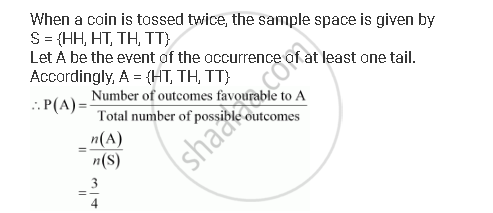CBSE (Arts) Class 11CBSE
Share

# A Coin is Tossed Twice, What is the Probability that at Least One Tail Occurs? - CBSE (Arts) Class 11 - Mathematics

ConceptAxiomatic Approach to Probability

#### Question

A coin is tossed twice, what is the probability that at least one tail occurs?

#### SolutionIs there an error in this question or solution?

#### Video TutorialsVIEW ALL 

Solution A Coin is Tossed Twice, What is the Probability that at Least One Tail Occurs? Concept: Axiomatic Approach to Probability.
S